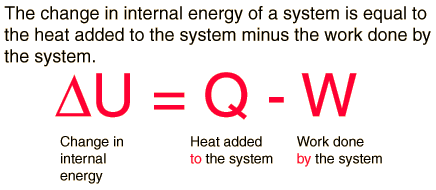# First Law of ThermodynamicsThe first law of thermodynamics is the application of the conservation of energy principle to heat and thermodynamic processes:The first law makes use of the key concepts of internal energy, heat, and system work. It is used extensively in the discussion of heat engines. The standard unit for all these quantities would be the joule, although they are sometimes expressed in calories or BTU.

It is typical for chemistry texts to write the first law as ΔU=Q+W. It is the same law, of course – the thermodynamic expression of the conservation of energy principle. It is just that W is defined as the work done on the system instead of work done by the system. In the context of physics, the common scenario is one of adding heat to a volume of gas and using the expansion of that gas to do work, as in the pushing down of a piston in an internal combustion engine. In the context of chemical reactions and process, it may be more common to deal with situations where work is done on the system rather than by it.

Second Law

The First Law of Thermodynamics, commonly known as the Law of Conservation of Matter, states that matter/energy cannot be created nor can it be destroyed. The quantity of matter/energy remains the same. It can change from solid to liquid to gas to plasma and back again, but the total amount of matter/energy in the universe remains constant.

The Second Law of Thermodynamics is commonly known as the Law of Increased Entropy. While quantity remains the same (First Law), the quality of matter/energy deteriorates gradually over time. How so? Usable energy is inevitably used for productivity, growth and repair. In the process, usable energy is converted into unusable energy. Thus, usable energy is irretrievably lost in the form of unusable energy.

“Entropy” is defined as a measure of unusable energy within a closed or isolated system (the universe for example). As usable energy decreases and unusable energy increases, “entropy” increases. Entropy is also a gauge of randomness or chaos within a closed system. As usable energy is irretrievably lost, disorganization, randomness and chaos increase.

http://illustramedia.com/